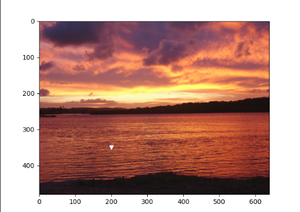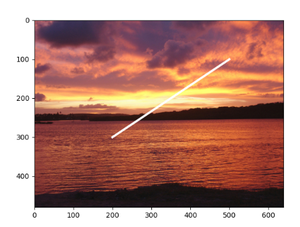GFG App
Open AppBrowser
Continue

# Plot a Point or a Line on an Image with Matplotlib

Prerequisites: Matplotlib

Matplotlib and its constituents support a lot of functionality. One such functionality is that we can draw a line or a point on an image using Matplotlib in python.

### Approach

• Import modules
• Plot the line or point on the image
• Display the plot/image.

Image Used:Implementation using the above approach on the given image is provided below:

Example 1 : Drawing a point on the image.

Attribute used: marker

It is used to define what marker type should the point be displayed in.

## Python3

 `from` `matplotlib ``import` `image ` `from` `matplotlib ``import` `pyplot as plt ` ` `  `# to read the image stored in the working directory ` `data ``=` `image.imread(``'sunset-1404452-640x480.jpg'``) ` ` `  `# to draw a point on co-ordinate (200,300) ` `plt.plot(``200``, ``350``, marker``=``'v'``, color``=``"white"``) ` `plt.imshow(data) ` `plt.show() `

Output:Example 2 : Draw a line on the image

To draw a line we will give the co-ordinates of two points in the plot function.

Attribute used: linewidth

Used to specify the width of the line.

## Python3

 `from` `matplotlib ``import` `image ` `from` `matplotlib ``import` `pyplot as plt ` ` `  `# to read the image stored in the working directory ` `data ``=` `image.imread(``'sunset-1404452-640x480.jpg'``) ` ` `  `# to draw a line from (200,300) to (500,100) ` `x ``=` `[``200``, ``500``] ` `y ``=` `[``300``, ``100``] ` `plt.plot(x, y, color``=``"white"``, linewidth``=``3``) ` `plt.imshow(data) ` `plt.show() `

Output:Example 3 : Draw two intersecting lines crossing each other to make X.

## Python3

 `from` `matplotlib ``import` `image ` `from` `matplotlib ``import` `pyplot as plt ` ` `  `# to read the image stored in the working directory ` `data ``=` `image.imread(``'sunset-1404452-640x480.jpg'``) ` ` `  `# to draw first line from (100,400) to (500,100) ` `# to draw second line from (150,100) to (450,400) ` `x1 ``=` `[``100``, ``500``] ` `y1 ``=` `[``400``, ``100``] ` `x2 ``=` `[``150``, ``450``] ` `y2 ``=` `[``100``, ``400``] ` `plt.plot(x1, y1, x2, y2, color``=``"white"``, linewidth``=``3``) ` `plt.axis(``'off'``) ` `plt.imshow(data) ` `plt.show() `

Output :My Personal Notes arrow_drop_up